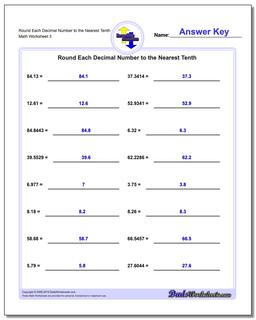# Math Worksheets: Rounding Numbers: Rounding Numbers: Round Each Decimal Number to the Nearest Tenth (Third Worksheet)## Round Each Decimal Number to the Nearest Tenth (Third Worksheet)

PropertyValue
DescriptionRound Each Decimal Number to the Nearest Tenth: Rounding decimal worksheets with decimal numbers where there may be significant digits to the left of the rounding place value. (Third Worksheet)
Resource TypeWorksheet